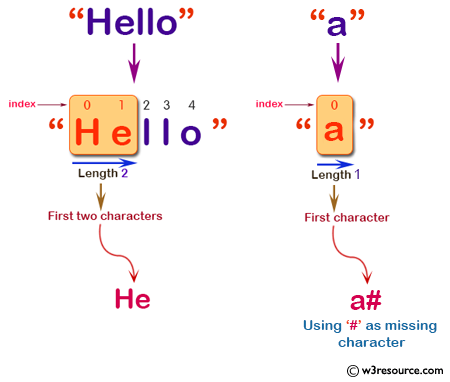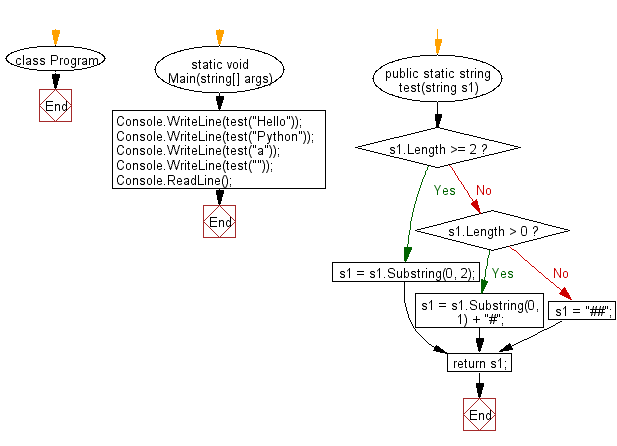﻿ C# - String of length 2, using first 2 elements of a string# C# Sharp Basic Algorithm Exercises: Create a new string of length 2, using first two characters of a given string

## C# Sharp Basic Algorithm: Exercise-76 with Solution

Write a C# Sharp program to create a new string of length 2, using first two characters of a given string. If the given string length is less than 2 use '#' as missing characters.

Pictorial Presentation:Sample Solution:-

C# Sharp Code:

``````using System;

namespace exercises
{
class Program
{
static void Main(string[] args)
{
Console.WriteLine(test("Hello"));
Console.WriteLine(test("Python"));
Console.WriteLine(test("a"));
Console.WriteLine(test(""));
}

public static string test(string s1)
{
if (s1.Length >= 2)
{
s1 = s1.Substring(0, 2);
}
else if (s1.Length > 0)
{
s1 = s1.Substring(0, 1) + "#";
}
else
{
s1 = "##";
}

return s1;
}
}
}
```
```

Sample Output:

```He
Py
a#
##```

Flowchart:C# Sharp Code Editor:

Improve this sample solution and post your code through Disqus

What is the difficulty level of this exercise?

Test your Programming skills with w3resource's quiz.

﻿